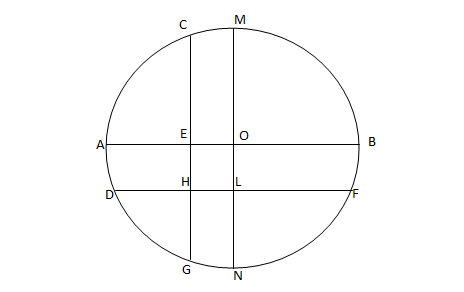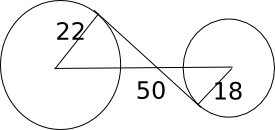0
185

Download Circle Questions for NMAT PDF – NMAT Fill in the blanks questions pdf by Cracku. Top 10 very important Circle Questions for NMAT based on asked questions in previous exam papers.

Take NMAT mock test

Question 1: What is the area of the minor segment formed by arc AB in a circle with center O, if the central angle of the arc is 36.87 degrees and the radius of the circle is 10 cm? ( Take sin 36.87 = 3/5).

a) 5 $cm^2$

b) 4 $cm^2$

c) 3 $cm^2$

d) None of the above

Question 2: What is the length of the arc AB in a circle whose radius is 14 cm and the angle subtended by the arc AB at a point P on the circumference is 30 degrees?

a) 50 cm

b) 88/3 cm

c) 22/3 cm

d) 44/3 cm

Question 3: In a circle with center O, arcs AB, BC, CD … HA are in AP. If the angle subtended by the arc HA at the center of the circle is 70 degrees, what is the angle subtended by the arc EF on the circumference of the circle?

a) 20 degrees

b) 42.24 degrees

c) 24.28 degrees

d) 30 degrees

Question 4: 2 chords AB and PQ are equidistant from the center O. The radius of the circle is 10 cm and the distance of chord AB from O is $10/\sqrt2$ cm. What is the ratio of the area of the minor sector formed by the arc AB to the area of the major sector formed by the arc PQ?

a) 1:3

b) 1:4

c) 2:3

d) Cannot be determined

Question 5: The length of the chord of a circle is 10 cm and the distance of the chord from the center is $5\sqrt3$ cm. What is the area of the minor segment subtended by the chord?

a) 13 $cm^2$

b) 9.08 $cm^2$

c) 12.2 $cm^2$

d) None of the above

Question 6: The length of the common chord of two circles of radii 15 cm and 20 cm, whose centres are 25 cm apart, is[CAT 2002]

a) 24 cm

b) 25 cm

c) 15 cm

d) 20 cm

Question 7: In the following figure, the diameter of the circle is 3 cm. AB and MN are two diameters such that MN is perpendicular to AB. In addition, CG is perpendicular to AB such that AE:EB = 1:2, and DF is perpendicular to MN such that NL:LM = 1:2. The length of DH in cm is[CAT 2005]

a) $2\sqrt2 – 1$

b) $(2\sqrt2 – 1)/2$

c) $(3\sqrt2 – 1)/2$

d) $(2\sqrt2 – 1)/3$

Question 8: A hexagon is inscribed in a circle of radius 10 cm. What is the area of the hexagon?

a) $150\sqrt{3} cm^2$

b) $150\sqrt{2} cm^2$

c) $100\sqrt{3} cm^2$

d) $100\sqrt{2} cm^2$

Question 9: What is the distance in cm between two parallel chords of lengths 32 cm and 24 cm in a circle of radius 20 cm?[CAT 2005]

a) 1 or 7

b) 2 or 14

c) 3 or 21

d) 4 or 28

Question 10: The distance between two circles of radius 22 cm and 18 cm is 50 cm. Find length of the transverse common tangent ?

a) 20 cm

b) 30 cm

c) 40 cm

d) 50 cm

sin 36.87 = 3/5

Area of the triangle = 1/2 * 10 * 10 * sin(36.87) = 50*3/5 = 30

Area of the sector = 36.87/360 * 22/7 * 10 * 10 = 32.18

So, the area of the minor segment = 2.18 $cm^2$

The angle subtended by the arc AB at the center of the circle is 60 degrees. The circumference of the circle is 2*22/7*14 = 88 cm
So, the length of the arc is 60/360 * 88 cm = 88/6 cm = 44/3 cm

Let the angle subteded by the first arc at the center be a, the angle subtended by the second arc at the center be (a+d) and so on.

So sum of the 8 arcs = n/2 * ( 2a + (n-1) d)=360° where n=8

So, 4(2a+7d) = 360 => 2a + 7d = 90

Also, angle subtended by HA at the center = a + 7d = 70

So, a = 20 and d = 50/7

Angle subtended by the arc EF at the center = a+4d = 20+200/7

So, the angle subtended at the circumference = 10+100/7 = 24.28 degrees

In triangle AOB, cos (AOC) = $\frac{\frac{10}{\sqrt{2}}}{10} = \frac{1}{\sqrt{2}}$ => angle AOC = 45. Similarly angle BOC=45. Now angle AOC+angle =angle AOB = 45+45 =90. Hence, AO is perpendicular to OB.

Similarly, angle POQ=90

The angle formed by minor sector of both the arcs will be 90 and the angle formed by the arcs of both the major sectors will be 360-90=270

For 360 degree the area = area of circle. Hence for 90 degree, the area of the minor sector is (area of the circle)/4  …(1)

So, the area of major sector of PQ = 270/360* area of the circle = 3*(area of the circle)/4  …..(2)

The required ratio = 1:3   (dividing 1 by 2)

The radius of the circle is $\sqrt{5^2 + (5\sqrt3)^2}$ = 10 cm
The angle subtended by the chord at the center is 30+30 = 60 degrees.
We have to find the area of sector AEB (the area enclosed by AEBDA).
So, the area of the sector = 60/360 * 22/7 * 10*10 = 2200/42 = 52.38
Area of the triangle is $1/2*10*5\sqrt3$ = $25\sqrt3$ = 43.30
So, the area of the segment is $9.08 cm^2$

The radii of both the circles and the line joining the centers of the two circles form a right angled triangle. So, the length of the common chord is twice the length of the altitude dropped from the vertex to the hypotenuse.

Let the altitude be h and let it divide the hypotenuse in two parts of length x and 25-x

So, $h^2 + x^2 = 15^2$ and $h^2 + (25-x)^2 = 20^2$

=> $225 – x^2 = 400 – x^2 + 50x – 625$

=> 50x = 450 => x = 9 and h = 12

So, the length of the common chord is 24 cm.

Let EO = x, So, AE = 3/2 – x

AE : EB = 1:2 => x = 1/2

So, EO = 1/2

Similarly, OL = 1/2

Now, EOLH is a parallelogram and EO = OL = 1/2.

So, OL = 1/2

In triangle DOL, DO = radius = 3/2 and OL = 1/2

So, DL = $\sqrt2$

=> DH = $(2\sqrt2 – 1)/2$

The length of the line joining the center of the hexagon to any of the vertices is same as the side of the hexagon. So, the side of the inscribed hexagon is equal to the radius of the circle = 10 cm. The area of the hexagon is $\sqrt{3}/4*10*10*6$ = $150\sqrt{3} cm^2$Length of the transverse common tangent = $\sqrt{50^2-(22+18)^2}=30$ cm